Problems of the Week

Contribute a problem

2018-06-04 Intermediate

There's a randomly ordered line of 15 forks and 15 spoons on the counter. Chef Nidhi only needs 5 forks and 5 spoons.

Can she always pick up exactly what she needs by selecting 10 consecutive utensils starting from somewhere in the line-up?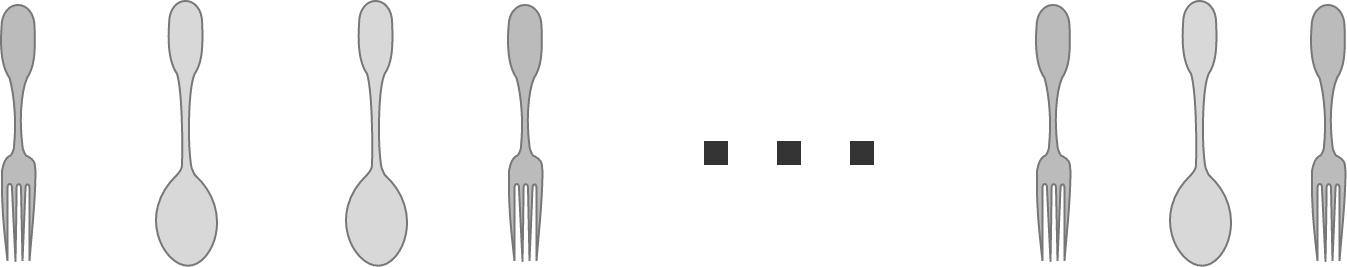The forks and spoons are not necessarily in this order. They can be in any order.

In basketball, when you're taking a shot, you generally want to aim for the middle of the basket to get a swish (when the ball doesn't touch the rim). But life isn't perfect and this doesn't always happen.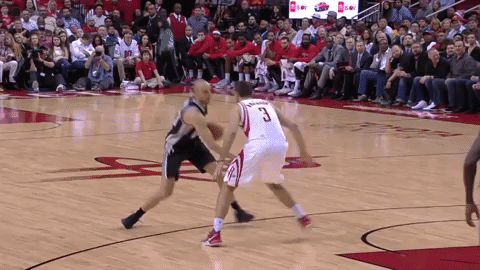If you want to maximize the chance that your shot goes in, even if you miss the swish and the ball bounces off the rim and/or backboard, what could you do with your shot besides aiming correctly?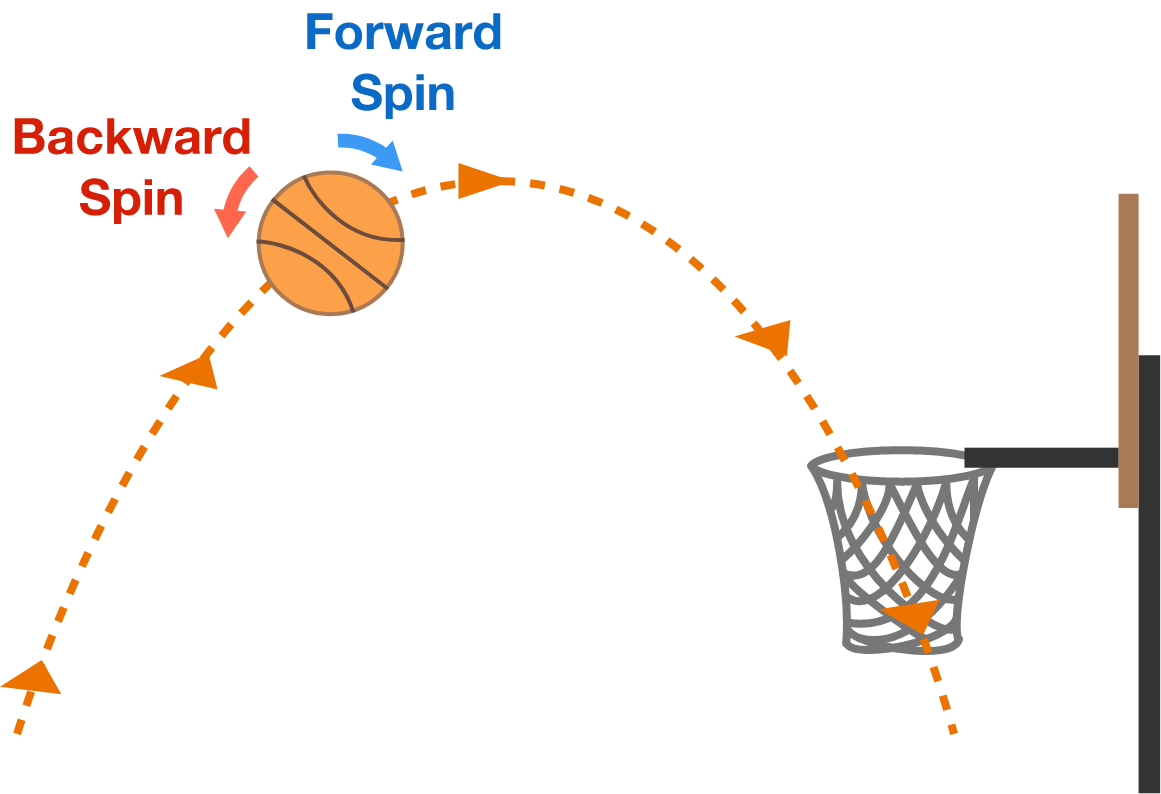A rectangular billboard $ABCD$ is illuminated by a lantern $E$ and casts a shadow in the $xy$-plane. The positions $(x,y,z)$ of the lantern and the billboard's vertices are $A = (0,0,0), \quad B = (0,3,0), \quad C = (0,3,2), \quad D = (0, 0 ,2), \quad E = (3, -1, 4).$ What is the area of the shadow?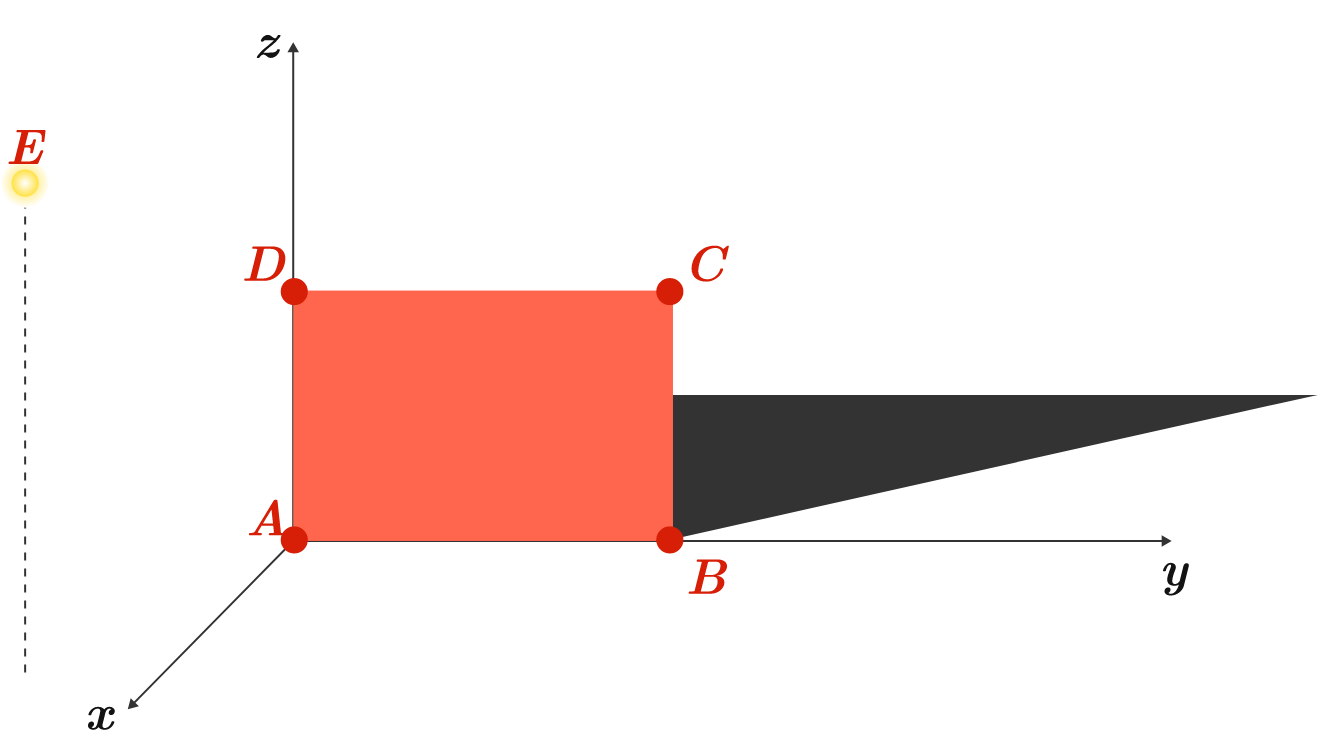Assumptions: The light source is a point and the billboard has zero thickness. The ground is the $xy$-plane.

A special type of chain pulley can be used to manually lift very heavy objects like car engines.

There are two pulleys, red (with radius 5) and blue (with radius 4), that move together and determine the maximum weight that can be lifted. Pulling on the slack part of the chain (black dots) wrapping around the pulleys lifts the box.

What is the mechanical advantage of this pulley? (That is, if I pull with a force of 1 Newton, how many times of upward force is applied on the brown box?)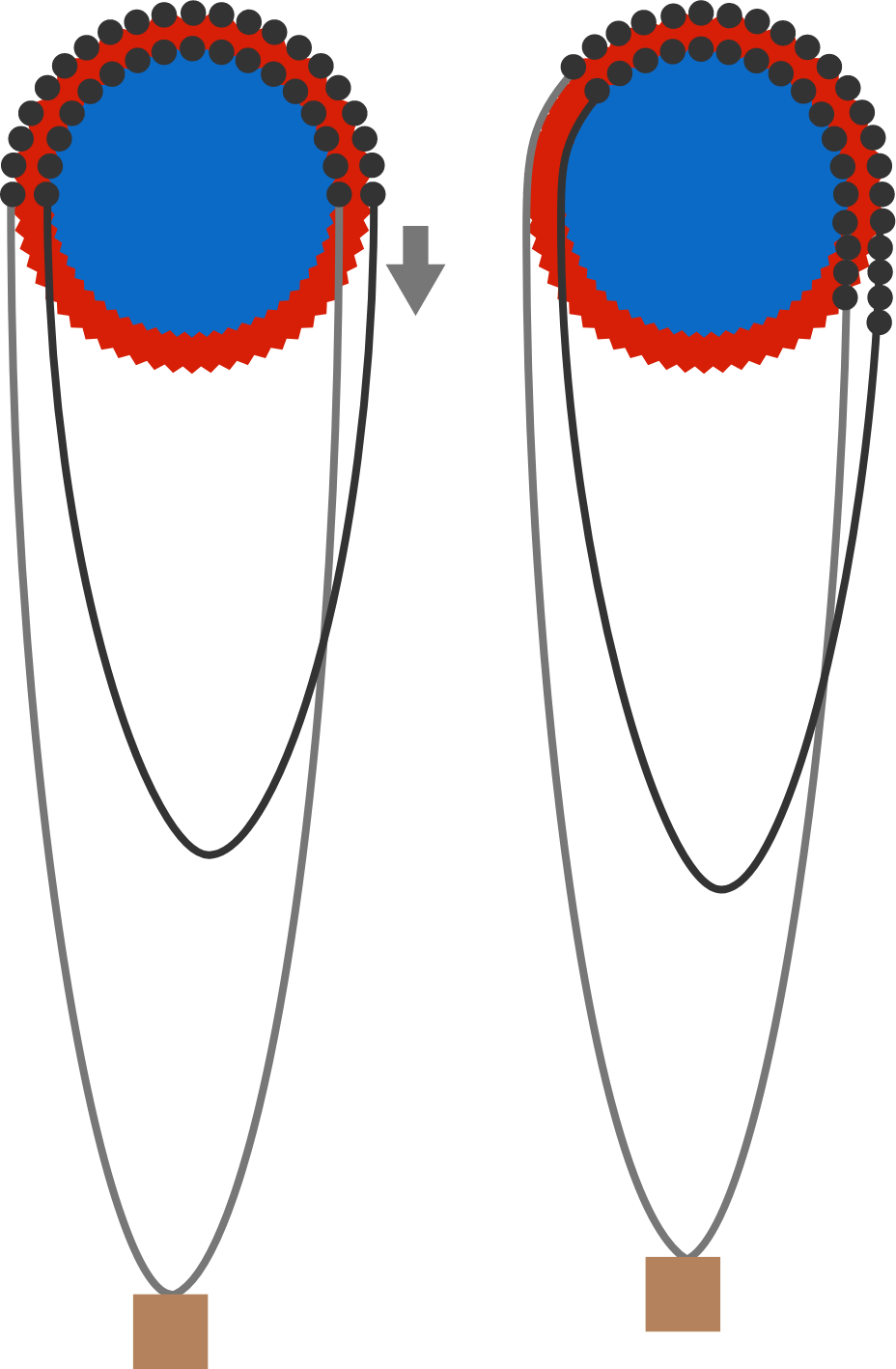Assumptions:

• Assume that the two lines that pull on the brown box are vertical (that is, there is no loss of mechanical advantage due to a bad angle).
• Assume that the pulleys and the chain are weightless and that there is no friction.

Alexandra, Bob, Chelsea, and Don are all logic experts who always tell the truth.

They each ate some numbers of apples: $a, b, c,$ and $d,$ respectively. They don't know the numbers the others ate, but they do know that each person ate at least 1 apple and that they ate 11 apples in total.

Alexandra says, “Bob, I don't know whether you ate more than I did. Did you?”
Bob replies, “I don’t know. Chelsea, I also don't know whether you ate more than I did. Did you?”
Chelsea replies, “I don’t know.”
Then Don says, "Oh, now I know how many each of us ate."

How many apples did each person eat? Give your answer as the concatenation of their numbers: $\overline{abcd}.$

Assume that the people all have perfect knowledge about each others' logical ability and honesty. That is, they all know that they are all logic experts that always tell the truth, and they all know that they know this, and so on.

×